## CGAL::rectangular_p_center_2

### Definition

The function rectangular_p_center_2 computes rectilinear p-centers of a planar point set, i.e. a set of p points such that the maximum minimal L-distance between both sets is minimized.

More formally the problem can be defined as follows.

Given a finite set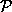of points, compute a point set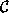with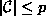such that the p-radius of,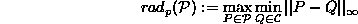is minimized. We can interpretas the best approximation (with respect to the given metric) forwith at most p points.

#include <CGAL/rectangular_p_center_2.h>

template < class ForwardIterator, class OutputIterator, class FT, class Traits >
OutputIterator
 rectangular_p_center_2 ( ForwardIterator f, ForwardIterator l, OutputIterator o, FT& r, int p, Traits t = Default_traits)

computes rectilinear p-centers for the point set described by the range [f, l), sets r to the corresponding p-radius, writes the at most p center points to o and returns the past-the-end iterator of this sequence.

Precondition

1. The range [f, l) is not empty.
2. 2p4.

The geometric types and operations to be used for the computation are specified by the traits class parameter t. This parameter can be omitted if ForwardIterator refers to a point type from the 2D-Kernel. In this case, a default traits class (Rectangular_p_center_default_traits_2<K>) is used.

Requirement

1. Either: (if no traits parameter is given) Value type of ForwardIterator is CGAL::Point_2<K> for some representation class K and FT is equivalent to K::FT,
2. Or: (if a traits parameter is specified) Traits is a model for RectangularPCenterTraits_2.
3. OutputIterator accepts the value type of ForwardIterator as value type.

### See Also

RectangularPCenterTraits_2
CGAL::Rectangular_p_center_default_traits_2<K>
CGAL::sorted_matrix_search

### Implementation

The runtime is linear for p{2,3} and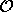(n · logn) for p = 4 where n is the number of input points. These runtimes are worst case optimal. The 3-center algorithm uses a prune-and-search technique described in [Hof99]. The 4-center implementation uses sorted matrix search [FJ83, FJ84] and fast algorithms for piercing rectangles [SW96].

### Example

The following code generates a random set of ten points and computes its two-centers.

```File: examples/Matrix_search/rectangular_p_center_2.cpp
```
```#include <CGAL/Cartesian.h>
#include <CGAL/point_generators_2.h>
#include <CGAL/rectangular_p_center_2.h>
#include <CGAL/IO/Ostream_iterator.h>
#include <CGAL/algorithm.h>
#include <iostream>
#include <algorithm>
#include <vector>

typedef double                                      FT;

struct Kernel : public CGAL::Cartesian<FT> {};

typedef Kernel::Point_2                             Point;
typedef std::vector<Point>                          Cont;
typedef CGAL::Random_points_in_square_2<Point>      Generator;
typedef CGAL::Ostream_iterator<Point,std::ostream>  OIterator;

int main()
{
int n = 10;
int p = 2;
OIterator cout_ip(std::cout);
CGAL::set_pretty_mode(std::cout);

Cont points;
CGAL::copy_n(Generator(1), n, std::back_inserter(points));
std::cout << "Generated Point Set:\n";
std::copy(points.begin(), points.end(), cout_ip);

FT p_radius;
std::cout << "\n\n" << p << "-centers:\n";
CGAL::rectangular_p_center_2(
points.begin(), points.end(), cout_ip, p_radius, 3);
std::cout << "\n\n" << p << "-radius = " << p_radius << std::endl;

return 0;
}
// ----------------------------------------------------------------------------
// ** EOF
// ----------------------------------------------------------------------------
```